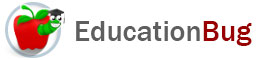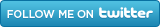PUBLIC SCHOOLS PRIVATE SCHOOLS SCHOOL DISTRICTS COLLEGES PUBLIC LIBRARIES JOBS BLOG RESOURCES
Career Education
Education Choices
Education Costs
Education History and General Information
Education Issues
Education Resources
Family Involvement in Education
Students w Special Needs
Subject Information and Homework Help
Teaching and Learning
Testing
By Types of Schools
By Specific Audiences
GeneralFractions

This article takes a look at fraction basics: what a fraction is, different ways to represent fractions, and how to work with fractions, turning them into decimals and percentages, for example, and converting fractions by finding the Least Common Denominator (LCD).

Fractions are ubiquitous in our lives, even in situations in which we may not consciously think of them. When we eat one piece of the leftover pizza and leave the rest for others, when we slice a sandwich before eating it, when we check the gas gauge in our vehicles, when we deal out cards for a game of â€œWarâ€ - in all these cases, weâ€™re working with fractions. For more about fractions, read the rest of this article.

What Are Fractions?

Fractions tell us about parts in relationship to a whole. That is, they give information about proportions or ratios: they do not tell absolute amounts. For example, saying that you have 1/8 of a pizza tells us about the portion in relation to the whole, but gives no information about the size of the piece. It could be from a personal size pizza with a 6-inch diameter or an extra-large pizza with a 24-inch diameter. The fraction doesnâ€™t convey that type of information.

Fractions have three parts: two numbers and a slash. Depending on the style, the slash may be horizontal or at an angle. Above the slash (and somewhat to the left, if the slash is at an angle) is the numerator. Below the slash (and somewhat to the right, if the slash is at an angle) is the denominator.

The denominator tells how many equal parts make a whole. The numerator tells how many of those parts are under consideration. The relationship of the numerator to the denominator is called a ratio. A ratio is a mathematical expression used to compare quantities relative to each other without reference to outside information.

So far, we have been speaking about fractions in relation to whole and parts of objects in the world. But it is important to realize that fractions arenâ€™t bound by the laws that govern such objects. For example, it is possible to have negative fractions, such as -2/3, and fractions that are more than a whole, such as 13/9. Fractions can also have a denominator that is much larger than any division we are likely to make, like 1/24,579. Fractions can also contain one or more variables, like this:

x/4                  y + z / 12              5/b

Different Representations of Fractions

Numbers that can be represented as fractions can also be represented as decimals and as percentages. This is easier to understand if we turn to a different consideration of fractions, one that considers it as a quotient, or the numerator divided by the denominator. For example, letâ€™s take the fraction Â½. If we rephrase it as a quotient, we would have:

1 Â¸ 2  =

and solving for that, we would get 0.5, which is the decimal expression of the fraction Â½. If we recall that a decimal can be expressed as a percentage by choosing to

• move the decimal point two places to the right